# Design of conditioning circuit for weak signal in through-casing resistivity logging

Design of conditioning circuit for weak signal in through-casing resistivity logging

Yongchun Hou

Department of Electron and Electricity Engineering, Baoji University of Arts and Science, Baoji 721016, China

Corresponding Author Email:
hyc0203@126.com
Page:
197-208
|
DOI:
https://doi.org/10.3166/EJEE.19.197-208
| |
Accepted:
| | Citation

OPEN ACCESS

Abstract:

The detection of extremely weak signals and suppression of interference are the key problems to be solved in through-casing formation resistivity logging. According to the characteristics of the signal to be measured, the signal conditioning circuit is designed. The principle and method for realization of power supply circuit, preamplifier circuit, middle-stage amplifier circuit and filter circuit are put forward. The result of measurement shows that conditioning circuit can accurately extract useful signals and suppress interference signals, and achieve the expected design goal.

Keywords:

extremely weak signals, through-casing resistivity logging, signal conditioning circuit, amplifier circuit, filter circuit

1. Introduction

The resistivity of the formation is measured by using the three-electrode method through measuring the voltage drop values generated by the excitation current on the casing (Wu et al., 2006; Kaufman & Wightman, 1993; Xu, 2016). The preamplifier is designed to amplify the voltage drop on the casing to provide high SNR signal for subsequent data acquisition. Because the casing resistance is very small, the logging signal of through-casing resistivity is weak, and its useful signal magnitude is as low as nV, considering the influence of noise, it is almost impossible to detect this weak signal directly (Li, 2012). Therefore, in the initial stage of signal detection, low noise pre-amplification is needed for this weak signal, and then the amplified signal is processed. The system demands that makes weak signal of nV enlarge to V, thus, expect the useful signal to be enlarged, the noise of ground, casing and itself circuit can be enlarged as well. And signal amplification circuit as the key terminal device of the whole logging system, its performance directly influences the whole logging system. The author designed through casing resistivity logging weak signal conditioning circuit, the key problems such as strong interference suppression and weak signal detection to be solved.

2. Through-casing resistivity logging signal characteristics

2.1. Frequency characteristics

The casing is a metal conductor on which electromagnetic waves will appear skin effects. With the signal characteristics increasing, the current approaches the inner surface of the

casing to make a few leakage currents to flow through the sleeve into the ground, it is difficult to measure the leakage current. Therefore, only when the frequency of excitation signal is low, it’s the better estimate leakage current to be measured on inner casing, then the formation resistivity can be calculated.

According to the formula of skin depth, it is set δc as skin depth of the casing,

${{\delta }_{c}}=\sqrt{\frac{2}{\omega \mu \sigma }}=\sqrt{\frac{2}{2\pi f\mu \sigma }}=\sqrt{\frac{1}{\pi f{{\mu }_{r}}{{\mu }_{0}}\sigma }}$     (1)

Known as μ0=4π×10-7(H/m), ρ=1/σ, generally relative permeability of steel casing in petroleum industry μr=50, resistivity ρ=2×10-7Ω·m, then the skin depth δc as:

${{\delta }_{c}}=3.18\times {{10}^{-2}}\sqrt{\frac{1}{f}}$      (2)

The Fig.1 shows the relationship between frequency of excitation signal and skin depth, with the frequency increasing, the skin depth reducing, thus the frequency should select lower, as long as the skin depth is greater than 2 times casing thickness, the measurement accuracy is high. For most sleeves, select form 1 Hz to 10 Hz signals to meet the requirements.

## 1.png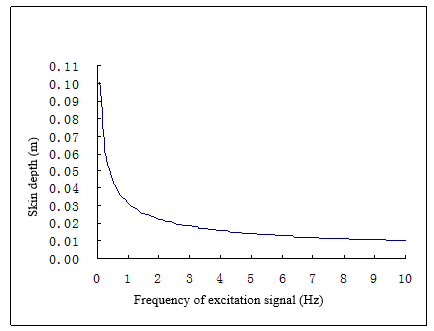Figure 1. Relationship between frequency of excitation signal and skin depth

2.2. Amplitude characteristics

The signal measurement by the casing logging is extremely weak at the nV level. Compared with other casing logging instruments, its signal 10 to 100 times lower than others. This is the technological difficulty of through casing formation resistivity logging weak signal detection.

The Fig.2 shows the actual signal magnitude measured by the casing resistivity logging, the through casing formation resistivity logging, direct measuring voltage values in μV (10-6 V) of inter-electrode. After difference the voltage in nV (10-9 V), the casing resistance in microhm (10-6Ω), leakage current in milliampere (10-3A).

## 2.png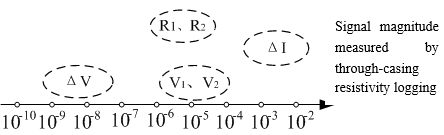Figure 2. The actual signal magnitude measured by the casing resistivity logging

3. System design

The structure design of the signal conditioning circuit (Fig. 3) is shown, including the power circuit design, preamplifier circuit design, filter circuit design, intermediate stage amplifier circuit design, isolated output circuit design.

## 3.pngFigure 3. Signal conditioning circuit

3.1. Power source

The ±5V power supply required for all modules of the signal conditioning board is generated by negative voltage linear regulator (LDO) TPS7A3001 (Zhang et al., 2009) and positive voltage linear regulator TPS7A4901 (Zhao et al., 2009). Both TPS7A3001 and TPS7A4901 are voltage linear regulators with extremely low noise, which can reduce the ripple noise of power supply and avoid the influence of power supply noise on the preamplifier circuit (Geng et al., 2011; Liu, 2014). The power supply circuit is shown in Fig.4 and 5.

## 4.pngFigure 4. -5V power circuit

Fig.5 shows a typical application circuit where the TPS7A3001 supplies -5V. Only the resistances of several external resistors are set can the power supply -5V. We can see from the characteristics of TPS7A3001 that the output voltage ranges from -1.184V to -33V, the resistors R3, R5 can be calculated by Equation (3).

${{R}_{3}}={{R}_{5}}(\frac{{{V}_{OUT}}}{{{V}_{REF}}}-1)$  (3)

## 5.pngFigure 5. +5V power circuit

The resistance in + 5V circuit is set in the same way as the -5V circuit, whereas the output voltage of TPS7A4901 ranges from + 1.194V to +33 V, R4, R6 can be calculated by the following formula (4):

${{R}_{4}}={{R}_{6}}(\frac{{{V}_{OUT}}}{{{V}_{REF}}}-1)$    (4)

The experiment shows that the ripple voltage output via the filter of the low dropout linear regulator is 30 μV, which meets the requirements of the circuit.

3.2. Low-noise preamplifier (LNP)

$F={{F}_{1}}+\frac{{{F}_{2}}-1}{{{K}_{1}}}+\frac{{{F}_{3}}-1}{{{K}_{1}}{{K}_{2}}}+...+\frac{{{F}_{M}}-1}{{{K}_{1}}{{K}_{2}}...{{K}_{M-1}}}$   (5)

It is known by the Folisi formula (Equation (5)) (Liu and Liu, 2014). The noise factors at all levels in the cascade amplifier produce different effects on the overall noise, the greater the effect is from the front amplifier. Therefore, the noise characteristics of the entire detection system depend on the noise factor of the preamplifier because the noise it generates is further amplified by subsequent amplifiers at all levels (Yan and Su, 2012). To design the weak signal conditioning circuit, the noise factor from the first stage amplifier must be lower enough.

Since the input of the first stage amplifier directly connects with the signal under detect where a common mode interference noise must be mixed. How to effectively filter out this noise is the primary task of the first stage amplifier. In this design, we adopt the low noise preamplifier LT1028 (Zhang, 2012) in the differential input mode. The LT1028 has a high common mode rejection ratio. The circuit in a differential input mode can greatly suppress the common mode signal, and amplify the differential mode signal. It is therefore better one to detect out the useful signals. The circuit for preamplifier (Figure 6) is shown.

## 6.png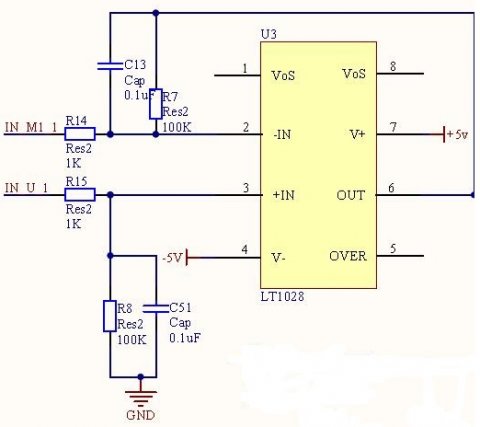Figure 6. Low-noise preamplifier

It can be seen from above figure that the theoretical amplification factor of low noise preamplifier is:

${{A}_{VF1}}=1+\frac{{{R}_{7}}}{{{R}_{14}}}\approx 101$   (6)

3.3. Filter circuit

Because noise and signal are amplified at the same time, it is not conducive to the work of subsequent amplification circuits. Therefore, the signal is filtered after being amplified by a preamplifier. To more effectively detect the useful signal, improve the signal to noise ratio (SNR) of the amplifier circuit, we not only adopt the low noise amplifier to reduce noise, but also further design a filter circuit to immunize the noise, improving the SNR at the output (Bao et al., 2013; Liu et al., 2016). This design procedure uses a first-order band-pass filter and second-order low-pass filter for noise immunization, gradually increases the SNR at the output.

As the signal under detect is ultralow frequent (ULF) at 1~10Hz. First we add a band-pass filter behind the preamplifier (Figure 7).

## 7.pngFigure 7. First-order band-pass filter

It can be seen from Fig.7, the low pass filter cutoff frequency of this band-pass filter is

${{f}_{L}}=\frac{1}{2\pi {{R}_{1}}{{C}_{1}}}\approx 14.109Hz$     (7)

The high pass filter cutoff frequency is

${{f}_{H}}=\frac{1}{2\pi {{R}_{2}}{{C}_{2}}}\approx 0.036Hz$    (8)

So says, the transmission band of this band-pass filter is 14.073Hz.

Since the system noise is rather complex, it is difficult to filter it out completely by the primary filter. The primary second-order, low-pass filter shall be added after the intermediate stage filter for optimizing noise immunization. Second-order low-pass filter (Figure 8) is shown, whose cut-off frequency is equal to that of band-pass filter, both are 14.109Hz.

## 8.pngFigure 8. Second-order low-pass filter

3.4. Intermediate stage amplifier

This design aims to detect out the weak signal on the casing pipe. As the amplitude of the signal on the casing pipe is at the microvolt level, we must amplify the amplitude of the signal under detect to the level that the acquisition circuit input requires. Here the intermediate stage amplifier circuit is designed.

According to the design idea of the pre-amplification electric circuit, the mid-amplification electric circuit adopts reverse proportional amplifier circuit. Since the noise comes mainly from high frequency components relative to useful signals, because of the larger amplitude, so in every lever amplification circuit output connect low pass filter circuit. In this way to the benefit of subsequent the amplifier circuit, and effectively improved SNR (Zhang et al., 2016). The mid-amplification electric circuit diagram is shown in Fig.9.

## 9.pngFigure 9. Intermediate stage amplifier

In Fig.9, the amplification factor of amplifier is mainly determined by R20, R12. The theoretical value is calculated as:

${{A}_{VF2}}=1+\frac{{{R}_{12}}}{{{R}_{20}}}\approx 101$          (9)

4. Debugging and test results of signal conditioning circuit

4.1. Debugging of signal conditioning circuit

The procedure for debugging the signal conditioning circuit is given as follows:

(1) Power debugging. Use a linear power supply MPS-3003LP-3 to connect + 5V terminal, -5V terminal and ground terminal to the circuit board, respectively, turn on the power. Observe whether the light-emitting diode displays normal, if not, immediately turn off the power, re-check whether the empty solder or missed weld exists on the circuit board, if so, repair it and try again until the light-emitting diode display normal. While a DC multimeter detects the capacitor C6 at the negative terminal is -5V, C8 is +5 V at the positive terminal, to determine the supply voltage of circuit board is normal. Note that the magnitude of the current is normal when turning on the power, it means normal if it does not change. Otherwise it is not normal, immediately turn off the power to prevent the circuit board burnout. If the current is too large, it exceeds the load capacity of the circuit board, the circuit board may be burned down.

(2) Debugging with the input signal. In the case of normal power supply, the signal is applied to the circuit board. The circuit designed in this paper includes two stages of amplifiers and three stages of filter. Theoretically the circuit magnification factor is calculated as 10000, and the signal frequency range is 1 ~ 10Hz. The amplifier circuit is debugged to verify the availability and stability of the circuit. First, two signals to be tested are available from the Ajilent 33220 signal source and the resistance attenuation network composed of four resistors (1K and 1Ω for every two respectively) (Dutta et al., 2012; Zhou et al., 2013). The signal amplitude of Ajilent 33220 is shifted from 10 mV to 200 mV in turn, i.e. 5uV~100uV applied to the input terminal of amplifier circuit, which is calculated based on the input voltage of the signal source divided by 2002. And then we use the oscilloscope to observe the output waveform of the, then we can see the output waveform frequency of receiver board is correct, but the amplitude lacks (inconsistent with theoretical value). This is because that the circuit adopts the passive filter, which has an attenuation effect on the signal amplitude (Wang et al., 2014). The actual magnification of the circuit board, therefore, can be calibrated based on the voltage amplitudes of the output and the input.

## 10.jpg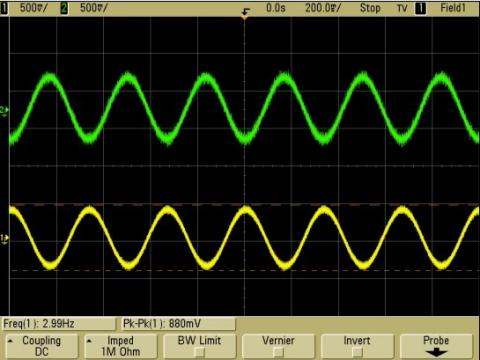Figure 10. Output wave of receiver board

Here the signal source is set to a sine wave with frequency of 3Hz and amplitude of 100mV, i.e. the equivalent input voltage of receiver board is 5.0E-05. Clip the oscilloscope at the output terminal of last stage, observe the oscilloscope waveform, as shown in Fig. 10.

It is deduced from the oscilloscope results that V1=3.16E-01V, V2=2.85E-01V. After many changes in the input and output voltage, the magnification factors in two channels are available, i.e. about F1=6330, F2=5690.

It can be seen from the above measurement results that this circuit behaves good performance. Then two performance indexes were inferred for the amplifier circuit:

(1) Amplification factor: 6330 x in channel 1; 5690 x in channel 2.

(2) The transmission band is 14.073Hz in width.

4.2. Weak signal voltage magnitude

Selecting three testing electrodes from casing, the mid-electrode as the common input. When the excitation current is 3.32A, the low noise amplifier to amplify two signals between the electrodes on the casing, after signal collection signal to using processing circuit is processed. The amplify circuit input voltage signals is MV. The weak signal voltage magnitude measuring result is shown in Fig.11.

## 11.png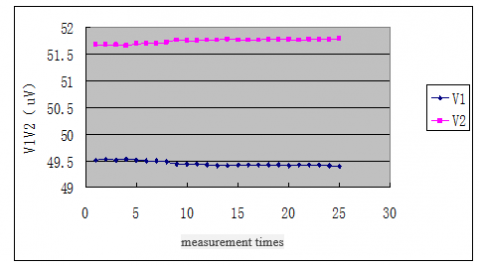Figure 11. Test results of weak signal

Experiment results show that the amplifier circuit designed could meet requires effectively and owns good filtering characteristics.

5. Conclusions

This paper makes a study on the principle and implementation of conditioning circuit for faint signal in through-casing resistivity logging. The test results show that the conditioning circuit can meet the design requirements, and its main performance indexes achieve the desired results.

Acknowledgment

The author gratefully acknowledges the Science and Technology Program of the Education Department of China’s Shaanxi Province (No. 16JK1044), the Science and Technology Program of Baoji University of Arts and Sciences (No. YK1512, 18JGYB50) for the research grant.

References

Bao D. Z., Liu J., Wang L. N. (2013). Development of TCFR cased-hole resistivity logging tool. Well Logging Technology, Vol. 37, No. 3, pp. 314-316.

Dutta S. M., Reiderman A., Schoonover L. G. (2012). New borehole transient electromagnetic system for reservoir monitoring. Petrophysics-SPWLA-Journal of Formation Evaluation and Reservoir Description, Vol. 53, No. 3, pp. 222.

Geng M., Liang H. Q., Cao X. D., Yin H. D., Zhang X. L. (2011). Design of signal conditioning circuits for cased-hole resistivity logging tool. Science Technology and Engineerong, Vol. 11, No. 6, pp. 1171-1175.

Kaufman A. A., Wightman W. E. (1993). A transmission-line model for electrical logging through casing. Geophysics, Vol. 58, No. 12, pp. 1739-1747. https://doi.org/10.1190/1.1443388

Li J., Sun J. M., Wang Z. K. (2012). On through casing resistivity logging. Well Logging Technology, Vol. 36, No. 1, pp. 63-67. https://doi.org/10.3969/j.issn.1004-1338.2012.01.013

Liu X., Wang J., Zou H. B., Zhang P. J., Yang W. (2016). Design and implementation of dynamic filtering circuit for the cross-hole logging tool. Petroleum Tubular Goods & Instruments, Vol. 2, No. 6, pp. 34-37.

Liu Y. (2014). Casing well logging signal processing methods. Xi'an: Xi'an Shiyou University.

Liu Y., Liu G. Q. (2014). Numerical simulation and analysis on the influence of casing inhomogeneity on through-casing resistivity logging response. Chinese Journal of Geophysics, Vol. 57, No. 4, pp. 1345-1355. https://doi.org/10.6038/cjg20140431

Wang H., Barker T., Chen K. (2014). Triaxial induction logging: theory, modeling, inversion and interpretation. SPE International Oil & Gas Conference and Exhibition, Beijing, pp. 347-351.

Wu Y. C., Zhang J. T., Yan Z. G. (2006). An overview of the logging technology of formation resistivity through casing. Petroleum Instruments, Vol. 20, No. 5, pp. 1-5. https://doi.org/10.3969/j.issn.1004-9134.2006.05.001

Xu X. F. (2016). Application of through casing resistivity logging in Gangxi oilfield. Petroleum Tubular Goods & Instruments, Vol. 2, No. 3, pp. 65-66, 69. 10.3969/j.issn.1004-9134.2016.03.017

Yan Z. G., Su J. (2012). Through-casing resistivity logging signal acquisition and processing techniques. Well Logging Technology, Vol. 36, No. 1, pp. 20-23. https://doi.org/10.4028/www.scientific.net/AMR.403-408.2659

Zhang J. T., Cui X., Xu K. (2016). Research on downhole instrument of cased hole resistivity logging system based on casing design. Modern Electronics Technique, Vol. 39, No. 7, pp. 27-129.

Zhang J. T., Huo F. F., Yan Z. G. (2009). Design of signal conditioning circuits for cased hole formation resistivity logging. Well Logging Technology, Vol. 33, No. 5, pp. 483-486. https://doi.org/10.1109/ITCS.2010.117

Zhang X. M. (2012). The acquisition theory and experiment of the weak signal on nV level of the cased hole resistivity logging. Xi'an: Xi'an Shiyou University.

Zhao X. L., Li S., Li K. (2009). Ultralow noise amplifier design in logging technology of formation resistivity through casing. World Well Logging Technology, Vol. 23, No. 2, pp. 10-11, 14.

Zhou J. H., Wang L., Yuan R. (2013). Analysis on effect of casing collar on formation resistivity logging through casing. Progress in Geophysics, Vol. 28, No. 1, pp. 421-426.